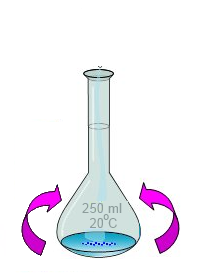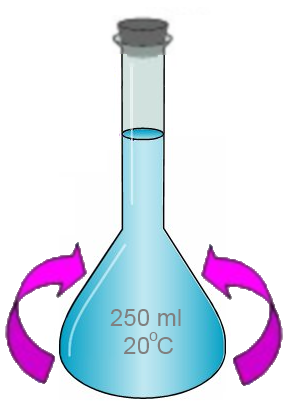Search:

# Preparation of a standard solution by dissolving

- Calculate the mass of solid which must be dissolved in the given volume. For example, to prepare of $250\; ml$ of a $0.2$ molar solution of sodium chloride, we need $n$ $=$ $[NaCl]\cdot V$ $=$ $0.2\cdot 0.25$ $=$ $0.05\; mol$, ie $m$ $=$ $n\cdot M$ $=$ $0.5\cdot 58.44$ $=$ $2.922\; g$ $NaCl$ - Weigh accurately that mass:- Introduce in a volumetric flask:- Rinse with distilled water into the volumetric flask:- Introduce distilled water:- Stir to dissolve the solid:- Fill distilled water to the mark:- Stopper the flask and stir:- The standard solution (the molarity is known) is ready to use !

Pictures taken from: →   Lycée Clemenceau de Reims